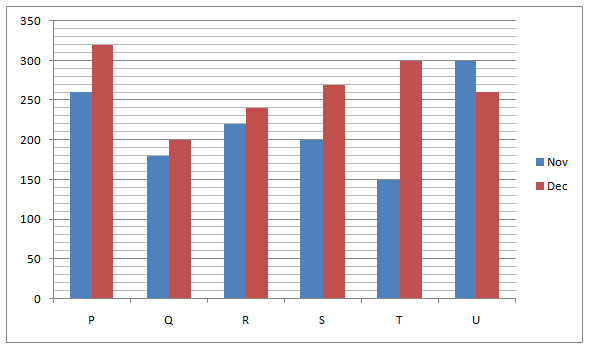# LIC AAO/SBI PO Prelims Quantitative Aptitude Questions 2019 (Day-06)

Dear Aspirants, Our IBPS Guide team is providing new series of Quantitative Aptitude Questions for LIC AAO/SBI PO 2019 so the aspirants can practice it on a daily basis. These questions are framed by our skilled experts after understanding your needs thoroughly. Aspirants can practice these new series questions daily to familiarize with the exact exam pattern and make your preparation effective.

[WpProQuiz 5547]

### Click Here for SBI PO Pre 2019 High-Quality Mocks Exactly on SBI Standard

Directions (Q. 1 – 5): What value should come in place of (?) in the following questions?

1) (2/5) of ? – (5/7) of (91/40) of 3648 = 28 % of 1850

a) 19450

b) 21360

c) 16115

d) 23785

e) None of these

2) 275 ÷ 11 + 1950 ÷ 13 + 1404 ÷ 9 = (?) % of 500

a) 66.2

b) 54.8

c) 72.6

d) 81.5

e) None of these

3) 27 % of 300 + 43 % of 400 – 7 % of 600 = ?

a) 187

b) 156

c) 243

d) 211

e) None of these

4) (9/28) ÷ (72/392) × 920 – 426 + 69 % of 1400 =?

a) 2860

b) 2150

c) 3380

d) 3520

e) None of these

5) 12 (¾)% of 2400 + 23 (½)% of 800 + 452 = 1125 ÷ 25 + 4 × ?

a) 726

b) 854

c) 618.5

d) 532.5

e) None of these

Directions (Q. 6 – 10): Study the following information carefully and answer the given questions:

The following line graph shows the total number of credit cards issued by 6 different banks in 2 different months in the year 2018.6) Find the average number of credit cards issued by all the given banks together in the month of Dec?

a) 280

b) 250

c) 265

d) 235

e) None of these

7) In which of the following bank has issued second lowest number of credit cards while comparing with both the months?

a) Bank T

b) Bank S

c) Bank Q

d) Bank P

e) None of these

8) Total number of credit cards issued by bank P is approximately what percentage of total number of credit cards issued by bank U in both the months?

a) 123 %

b) 92 %

c) 78 %

d) 104 %

e) 135 %

9) Find the difference between the average number of credit cards issued by bank P, R and T together in the month of Nov to that of the bank P, Q and U together in the month of Dec?

a) 90

b) 50

c) 100

d) 70

e) None of these

10) Total number of credit cards issued by bank Q and T together is approximately what percentage more/less than the total number of credit cards issued by bank R and S together in both the months?

a) 24 % less

b) 32 % more

c) 11 % less

d) 24 % more

e) 11 % more

(2/5)*x – (5/7) * (91/40) * 3648 = (28/100)*1850

(2x/5) – 5928 = 518

(2x/5) = 518 + 5928

(2x/5) = 6446

X = 6446*(5/2) = 16115

(275/11) + (1950/13) + (1404/9) = (x/100)*500

25 + 150 + 156 = 5x

331= 5x

X = 331/5 = 66.2

27 % of 300 + 43 % of 400 – 7 % of 600 = x

X = (27/100)*300 + (43/100)*400 – (7/100)*600

X = 81 + 172 – 42

X = 211

(9/28)*(392/72)*920 – 426 + (69/100)*1400 = x

X = 1610 – 426 + 966

X = 2150

(51/400)*2400 + (47/200)*800 + 2025 = (1125/25) + 4x

306 + 188 + 2025 – 45 = 4x

2474 = 4x

X = 2474/4 = 618.5

The average number of credit cards issued by all the given banks together in the month of Dec

= > (320 + 200 + 240 + 270 + 300 + 260)/6

= > 1590/6 = 265

Bank P = > 260 + 320 = 580

Bank Q = > 180 + 200 = 380

Bank R = > 220 + 240 = 460

Bank S = > 200 + 270 = 470

Bank T = > 150 + 300 = 450

Bank U = > 300 + 260 = 560

Bank T has issued second lowest number of credit cards.

Total number of credit cards issued by bank P in both the months

= > 260 + 320 = 580

Total number of credit cards issued by bank U in both the months

= > 300 + 260 = 560

Required % = (580/560)*100 = 103.57 % = 104 %

The average number of credit cards issued by bank P, R and T together in the month of Nov

= > (260 + 220 + 150)/3 = 630/3 = 210

The average number of credit cards issued by bank P, Q and U together in the month of Dec

= > (320 + 200 + 260)/3 = 780/3 = 260

Required difference = 260 – 210 = 50

Total number of credit cards issued by bank Q and T together in both the months

= > 180 + 200 + 150 + 300 = 830

Total number of credit cards issued by bank R and S together in both the months

= > 220 + 240 + 200 + 270 = 930

Required % = [(930 – 830)/930]*100 = 10.75 % = 11 % less